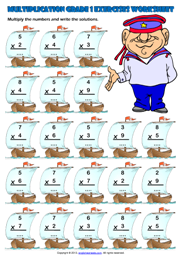Home Maths Main Page

# Single Digit Multiplication Maths Exercise Worksheet

A fun multiplication exercises maths worksheet for grade 1 (first grade) students and kids with ships and sailor theme. Multiply the numbers and write the solutions. (Vertical Multiplication Worksheet for kids)Next Worksheet Grade 1 Multiplication Main Page Home